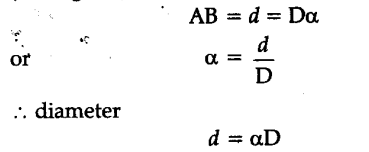# Give a method to determine the angular size of a planet

Size or diameter of a planet can be measured with the help of parallax method. Distance D of the planet from earth is measured with the help of
relation D = b/\theta where b is the distance between observatories on earth and \theta is the angle between two directions along which planet was viewed from two observations.
Let d be the diameter of planet and a the angle subtended by the diameter d at a point E on earth.
then a being very small,d/D < < 1. Let AB be an arc
(of length d) of a circle with centre E. Then distance1 Like